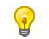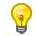# PHP 变量

 PHP 语法 本章完

# PHP 变量

## 实例

<?php \$x=5; \$y=6; \$z=\$x+\$y; echo \$z; ?>

运行预览 »

## 与代数类似

x=5
y=6
z=x+y变量是用于存储数据的容器。

## PHP 变量

PHP 变量规则：

• 变量以 \$ 符号开始，后面跟着变量的名称
• 变量名必须以字母或者下划线字符开始
• 变量名只能包含字母数字字符以及下划线（A-z、0-9 和 _ ）
• 变量名不能包含空格
• 变量名是区分大小写的（\$y 和 \$Y 是两个不同的变量）PHP 语句和 PHP 变量都是区分大小写的。

PHP 没有声明变量的命令。

## 实例

<?php \$txt="Hello world!"; \$x=5; \$y=10.5; ?>

运行预览 »

## PHP 是一门弱类型语言

PHP 会根据变量的值，自动把变量转换为正确的数据类型。

PHP 有四种不同的变量作用域：

• local
• global
• static
• parameter

## 实例

<?php
\$x
=5// 全局变量

function myTest()
{

\$y=10// 局部变量

echo "<p>测试函数内变量:<p>";
echo
"变量 x 为: \$x";
echo
"<br>";
echo
"变量 y 为: \$y";

myTest();

echo
"<p>测试函数外变量:<p>";
echo
"变量 x 为: \$x";
echo
"<br>";
echo
"变量 y 为: \$y";
?>

运行预览 »你可以在不同函数中使用相同的变量名称，因为这些函数内定义的变量名是局部变量，只作用于该函数内。

## PHP global 关键字

global 关键字用于函数内访问全局变量。

## 实例

<?php \$x=5; \$y=10; function myTest() { global \$x,\$y; \$y=\$x+\$y; } myTest(); echo \$y; // 输出 15 ?>

运行预览 »

PHP 将所有全局变量存储在一个名为 \$GLOBALS[index] 的数组中。 index 保存变量的名称。这个数组可以在函数内部访问，也可以直接用来更新全局变量。

## 实例

<?php \$x=5; \$y=10; function myTest() { \$GLOBALS['y']=\$GLOBALS['x']+\$GLOBALS['y']; } myTest(); echo \$y; ?>

## 实例

<?php function myTest() { static \$x=0; echo \$x; \$x++; echo PHP_EOL; // 换行符 } myTest(); myTest(); myTest(); ?>

运行预览 »

## 实例

<?php function myTest(\$x) { echo \$x; } myTest(5); ?>

 PHP 语法 本章完